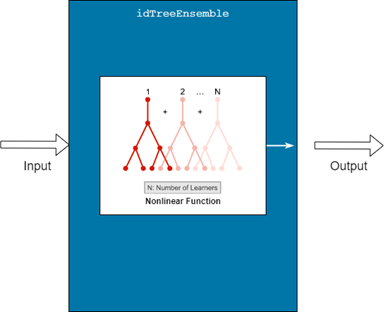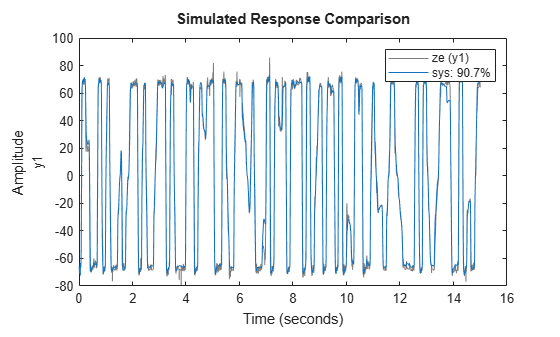# idTreeEnsemble

Decision tree ensemble mapping function for nonlinear ARX models (requires Statistics and Machine Learning Toolbox)

## Description

An `idTreeEnsemble` object implements a decision tree ensemble model, and is a nonlinear mapping function for estimating nonlinear ARX models. This mapping object incorporates regression tree ensembles that the mapping function creates using Statistics and Machine Learning Toolbox™. Unlike most other mapping objects for `idnlarx` models, which typically contain offset, linear, and nonlinear components, the `idTreeEnsemble` model contains only a nonlinear component.Mathematically, the `idTreeEnsemble` object maps m inputs x(t) = [x1(t),x2(t),…,xm(t)]T to a scalar output y(t) using a decision tree regression ensemble model.

Here:

• x(t) is an m-by-1 vector of inputs, or regressors.

• y(t) is the scalar output.

For more information about creating regression tree ensembles, see `fitrensemble` (Statistics and Machine Learning Toolbox).

Use `idTreeEnsemble` as the value of the `OutputFcn` property of an `idnlarx` model. For example, specify `idTreeEnsemble` when you estimate an `idnlarx` model with the following command.

`sys = nlarx(data,regressors,idTreeEnsemble)`
When `nlarx` estimates the model, it essentially estimates the parameters of the `idTreeEnsemble` object.

You can configure the `idTreeEnsemble` function to set options and fix parameters. To modify the estimation options, set the option property in `E.EstimationOptions`, where `E` is the `idTreeEnsemble` object. For example, to change the fit method to `'lsboost-resampled'`, use ```E.EstimationOptions.FitMethod = 'lsboost-resampled'```. To fix the values of an existing estimated `idTreeEnsemble` during subsequent `nlarx` estimations, set the `Free` property to `false`. To apply parallel processing, set `E.EstimationOptions.UseParallel` to `true`. Use `evaluate` to compute the output of the function for a given vector of regressor inputs.

## Creation

### Syntax

``E = idTreeEnsemble``
``E = idTreeEnsemble(fitmethod)``

### Description

example

````E = idTreeEnsemble` creates an empty `idTreeEnsemble` object `E` with the default estimation fit method of `'bag'`. The number of regressor inputs is determined during model estimation and the number of `idTreeEnsemble` outputs is 1.```
````E = idTreeEnsemble(fitmethod)` sets the ensemble estimation method to the value in `fitmethod`.```

### Input Arguments

expand all

Method to use for estimating the parameters of the `idTreeEnsemble` model, specified as `'bag'`, `'lsboost-reweighted'`, or `'lsboost-resampled'`.

This argument sets the property `E.EstimationOptions.FitMethod`. For more information, see `Estimation Options`.

## Properties

expand all

Input signal names for the inputs to the mapping object, specified as a 1-by-m cell array, where m is the number of input signals. This property is determined during estimation.

Output signal name for the output of the mapping object, specified as a 1-by-1 cell array. This property is determined during estimation.

Option to update the parameters of `RegressionEnsembleModel` during nonlinear ARX model estimation, specified as `true` or `false`. When `free` is `true`, the estimation process updates the ensemble model when it estimates the `idnlarx` model that contains it. When `free` is `false`, the ensemble model is fixed during estimation. Setting `free` to `false` is useful when you are using a previously estimated ensemble model as a mapping function for `nlarx`.

Estimation options for the `idTreeEnsemble` model, specified as follows. For more information on any of these options, see the corresponding name-value argument in `fitrensemble` (Statistics and Machine Learning Toolbox).

Main OptionDescription
`FitMethod`

Method to use for estimating the parameters of the `idTreeEnsemble` model, specified as one of the items in the following table.

Option Description
`'bag'`

Bagging (bootstrap aggregation) (default)

`'lsboost-reweighted'`

Least-squares boosting with reweighting

`'lsboost-resampled'`

Least-squares boosting with resampling

`Learners`

Options that control the estimation of individual regression trees (weak learners) in the ensemble, specified as described in the following table. For more information on these properties, see the corresponding argument descriptions in `templateTree` (Statistics and Machine Learning Toolbox).

OptionDescriptionDefault
`MaxNumSplits`Maximum number of decision splits, or branch nodes, per tree, specified as `'auto'` or a positive integer.`'auto'`
`MergeLeaves`Option to merge leaves that originate from the same parent node and that provide a sum of risk values greater than or equal to the risk associated with the parent node, specified as `'on'` or `'off'`. Node risk is defined as the node error weighted by the node probability.`'off'`
`MinLeafSize`Minimum number of observations per leaf, specified as positive integer.`5`
`PredictorSelection`

Algorithm used to select the best split predictor at each node, specified as one of the following:

• `'allsplits'`

• `'curvature'`

• `'interaction-curvature'`

For more information on these choices, see the corresponding argument in `templateTree` (Statistics and Machine Learning Toolbox).

`'allsplits'`
`Prune`Flag to estimate the optimal sequence of pruned subtrees, specified as `'off'` or `'on'`.`'off'`
`QuadraticErrorTolerance`Quadratic error tolerance per node, specified as a positive scalar. A regression tree stops splitting nodes when the weighted mean squared error per node drops below``` QuadraticErrorTolerance```*ε, where ε is the weighted mean squared error of all n responses computed before growing the decision tree.`1e-6`
`LearnRate`Learning rate for shrinkage, specified as a numerical scalar in the interval (0,1]. To train an ensemble using shrinkage, set `LearnRate` to a value less than 1. For example, `0.1` is a popular choice. Training an ensemble using shrinkage requires more learning iterations, but can achieve better accuracy. The default value is `1`.
`NumLearningCycles`Number of ensemble learning cycles, specified as a positive integer. The default value is `100`.
`ObservationWeights`

`ObservationWeights` — Observation weights, specified as `[]` or as a numeric column vector of length n, where n is the number of observations. The software weights each observation with the corresponding value in `ObservationWeights`. When `ObservationWeights` is set to `[]`, all observations get equal weight. The default value is `[]`.

`ResampleData`

`ResampleData` — Option to resample the data, specified as `'on'` (default) or `'off'`.

• If `FitMethod` is set to `'bag'`, then `ResampleData` must be set to `'on'`.

• If `FitMethod` is set to `'lsboost-reweighted'`, then `ResampleData` has no effect.

`ResampleFraction`

`ResampleFraction` — Fraction of training set to resample, specified as a positive scalar in (0,1].

• If `FitMethod` is set to `'lsboost-reweighted'`, then `ResampleFraction` has no effect.

`ReplaceData`

`ReplaceData` — Option to sample with replacement, specified as `'on'` (default) or `'off'`. This property has an effect only if either `FitMethod` is set to `'bag'` or `ResampleData` is set to `'on'` and `FitMethod` is set to `'lsboost-resampled'` .

`Regularize `

`Regularize` — Option to find optimal weights for learners, specified as `'on'` (default) or `'off'`.

`RegularizeOptions`

`RegularizeOptions` — Options for regularization, specified as described in the following table. The software applies these options when `Regularize` is `'on'`. For more information on these options, see the corresponding arguments in `regularize` (Statistics and Machine Learning Toolbox).

Option Description
`'Lambda'`

Lasso Penalty

Equivalent to `lambda` argument in `regularize` (Statistics and Machine Learning Toolbox).

`'MaxIterations'`

Maximum iterations for lasso search.

Equivalent to `maxiter` argument in `regularize`.

The default value is 1000.

`'NumPasses'`

Maximum number of passes for lasso.

Equivalent to `maxiter` argument in `regularize`.

The default value is 10.

`'RelativeTolerance'`

Relative tolerance on the regularized loss for lasso.

Equivalent to `reltol` argument in `regularize`.

The default value is 1e-3.

`Shrink `

`Shrink` — Option to prune ensemble and return a compact version, specified as `'off'` (default) or `'on'`.

`ShrinkOptions`

`ShrinkOptions` — Options for `shrink`, specified as described in the following table. The software applies these options when `Shrink` is `'on'`. For more information on these options, see the corresponding arguments in `shrink` (Statistics and Machine Learning Toolbox).

Option Description
`'Lambda'`

Lasso Penalty. Do not specify if `Regularize` is `true`.

Equivalent to `lambda` argument in `shrink` (Statistics and Machine Learning Toolbox).

The default value is `[]`.

`'Threshold'`

Lower cutoff on weights for weak learners.

Equivalent to `threshold` argument in `shrink`.

The default value is `0`.

`UseParallel`Option to use parallel computations for model training and response computation, specified as `false` (default) or `true`. Setting `UseParallel` to `true` is especially useful when you have a large ensemble, as the software can perform the computations for the individual regression trees in parallel. This option requires Parallel Computing Toolbox™.

## Examples

collapse all

Load the data `mrdamper`. This data contains damping force (`F`) and velocity (`V`) information for a fluid damper, with a sample time of `Ts`.

`load(fullfile(matlabroot,'toolbox','ident','iddemos','data','mrdamper'))`

Create an `iddata` object `data` that uses `F` as the output and `V` as the input. Divide `data` into estimation and validation data sets `ze` and `zv`.

```data = iddata(F,V,Ts); ze = data(1:3000); zv = data(3001:end);```

Create an `idTreeEnsemble` mapping object `E` with default settings.

`E = idTreeEnsemble;`

Estimate a nonlinear ARX model `sys` that uses `E` for the output function.

`sys = nlarx(ze,[16 16 0],E);`

The model stores the estimated mapping object in the property `sys.OutputFcn`.

`sys.OutputFcn`
```ans = Regression Tree Ensemble Inputs: y1(t-1), y1(t-2), y1(t-3), y1(t-4), y1(t-5), y1(t-6), y1(t-7), y1(t-8), y1(t-9), y1(t-10), y1(t-11), y1(t-12), y1(t-13), y1(t-14), y1(t-15), y1(t-16), u1(t), u1(t-1), u1(t-2), u1(t-3), u1(t-4), u1(t-5), u1(t-6), u1(t-7), u1(t-8), u1(t-9), u1(t-10), u1(t-11), u1(t-12), u1(t-13), u1(t-14), u1(t-15) Output: y1(t) Nonlinear Function: Bagged Regression Tree Ensemble Inputs: {1×32 cell} Outputs: {'y1(t)'} Free: 1 EstimationOptions: 'Estimation option set' ```

Compare the model simulated output to the estimation data output.

`compare(ze,sys)`Compare the model simulated output to the validation data output.

`compare(zv,sys)``sys` shows a good fit to both the estimation data and the validation data.

## Version History

Introduced in R2021b

expand all

Behavior changed in R2022a